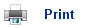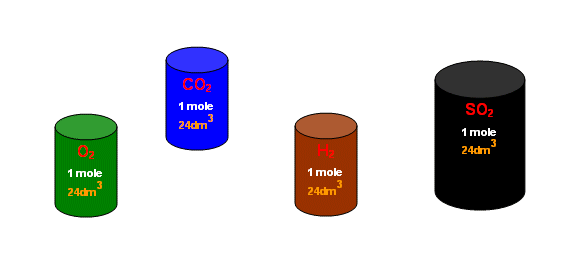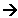# Volumes of Gases

• The number of molecules in one mole of gas is always 6 x 1023 (Avagadro's constant).
• The molecules of a gas are extremely spread out, and so the size of them is negligible compared to the total volume the gas occupies.
• So one mole of gas always occupies the same volume, and at standard conditions (1 atmosphere, 298K) that volume is 24dm3.• We can use this idea of molar volumes to work out the volumes of gases involved in a chemical reaction. For example,
Ammonia is produced from Hydrogen and Nitrogen:

N2(g) + 3H2(g)2NH3

From the equation, 1 mole of Nitrogen reacts with 3 moles of Hydrogen to produce 2 moles of Ammonia.

So, using this idea:

(1 x 22.4dm3) N2(g) + (3 x 22.4dm3) H2(g)(2 x 22.4dm3) NH3(g)

So if we had 10dm3 of Nitrogen, it would react with 30dm3 of Hydrogen to form 20dm3 of Ammonia (standard conditions).

## Useful books for revision:

Revise AS Chemistry for Salters (Written by experienced examiners and teachers of Salter's chemistry)Revise AS Chemistry for Salters (OCR) (Salters Advanced Chemistry)Home